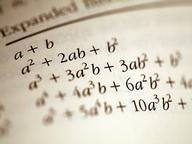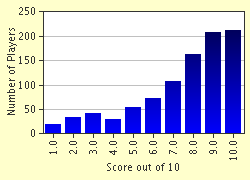FREE! Click here to Join FunTrivia. Thousands of games, quizzes, and lots more!# Polynomial Factoring Madness! Trivia Quiz

### This is a basic quiz on factoring all types of polynomials to their simplest form. Note that when an ^ comes before a number, like y^2, it means "to the power of." Hint: Always look for common factors first! Good luck!

A multiple-choice quiz by XxHarryxX. Estimated time: 3 mins.

Author
XxHarryxX
Time
3 mins
Type
Multiple Choice
Quiz #
202,033
Updated
Feb 24 23
# Qns
10
Difficulty
Average
Avg Score
7 / 10
Plays
4398
Last 3 plays: AdamM7 (10/10), Guest 41 (4/10), Guest 94 (4/10).
This quiz has 2 formats: you can play it as a or as shown below.
Scroll down to the bottom for the answer key.
1. Factor 5(x^3)(y^2)(z) + 100(x^2)(y^3)(z^2) by factoring out their greatest common factor. NOTE: The parentheses have been added to distinguish terms from each other. Factor as normal. Hint

5(x^3y^2z + 20x^2y^3z^2)
5xyz(x^2y + 20xy^2z)
(5(x^2)(y^2)(z))(x + 20yz)
5x^5y^5z^3(x + 20y)

#### NEXT>

2. Factor the polynomial 4x^2 - 25, using the difference of two perfect squares. Hint

(4x + 5)(4x - 5)
(2x + 5)(2x - 5)
(2x^2 + 5)(2x^2 - 5)
(2x + 25)(2x - 25)

#### NEXT>

3. Completely factor 2x^2 - 50. Hint

2(x^2 - 25)
2(x + 5)(x - 5)
(2x - 10)(x + 5)
x^2 - 25

#### NEXT>

4. Factor the perfect square polynomial 25x^2 + 90x + 81. Hint

none of these
(25x + 9)(x + 9)
(5x + 9)^2
(25x + 1)(x + 81)

#### NEXT>

5. Factor a^2 + 11a + 24. Hint

(a + 1)(a + 24)
(a + 4)(a + 6)
(a + 2)(a + 12)
(a + 8)(a + 3)

#### NEXT>

6. Factor the trinomial b^2 + 6b - 16. Hint

(b + 4)(b - 4)
(b + 8)(b - 2)
(b - 8)(b + 2)
(b + 16)(b - 1)

#### NEXT>

7. Completely factor 4b^2 + 16b + 16. Hint

none of these
(4b + 8)(b + 2)
4(b + 2)^2
(2b + 4)^2

#### NEXT>

8. Factor 3x^2 + 14x + 15. Hint

(3x + 5)(x + 3)
(3x + 3)(x + 5)
(1.5x + 7.5)^2
none of these

#### NEXT>

9. Factor the polynomial 12x^2 + x - 35. Hint

none of these
(12x + 35)( x - 1)
(4x + 7)(3x - 5)
(4x - 7)(3x + 5)

#### NEXT>

10. To wrap this quiz up, completely factor the following polynomial:
308x^2 + 161x + 21.
Hint

(28x + 7)(11x + 3)
none of these
(4x + 1)(77x + 21)
7(4x + 1)(11x + 3)

 (Optional) Create a Free FunTrivia ID to save the points you are about to earn:Select a User ID:Choose a Password:Your Email:

Most Recent Scores
Sep 23 2023 : AdamM7: 10/10
Sep 19 2023 : Guest 41: 4/10
Aug 25 2023 : Guest 94: 4/10
Aug 20 2023 : emmal2000uk: 5/10

Score DistributionQuiz Answer Key and Fun Facts
1. Factor 5(x^3)(y^2)(z) + 100(x^2)(y^3)(z^2) by factoring out their greatest common factor. NOTE: The parentheses have been added to distinguish terms from each other. Factor as normal.

Find the GCF of your like terms. The GCF of 5 and 100 is 5. The GCF of x^3 and x^2 is x^2. The GCF of y^2 and y^3 is y^2. The GCF of z and z^2. So, your total GCF is 5x^2y^2z. You divide each term by that and are left with x and 20yz. You write it in the form
5x^2y^2z(x + 20yz). If you distribute 5(x^2)(y^2)(z) to x and 20yz, you have your original answer, 5x^3y^2z + 100x^2y^3z^2.
2. Factor the polynomial 4x^2 - 25, using the difference of two perfect squares.

Answer: (2x + 5)(2x - 5)

Both terms are perfect squares. The square root of 4x^2 is 2x, and the square root of 25 is 5. You place one of each term in two sets of parentheses, and place an addition sign in the middle of one and a subtraction sign in the middle of another. The reason a problem like this is factored this way is when you use the distributive property to multiply it out, you get 4x^2 + 10x - 10x - 25. Your +10x and -10x cancel out to 0x, which means there is no need to write the 0x in the problem.
3. Completely factor 2x^2 - 50.

Answer: 2(x + 5)(x - 5)

Looking at the original problem, you notice a common factor of two in both terms. Factor it out now, to save yourself later trouble. You now have 2(x^2 - 25). Looking at the x^2 - 25, you notice both terms are perfect squares and, using the difference of two perfect squares technique, factor it further down to (x+5)(x-5). Your final answer would be 2(x+5)(x-5).
4. Factor the perfect square polynomial 25x^2 + 90x + 81.

Looking at your first and last terms, you notice they are both perfect squares. You can place their square roots in parentheses and add them together in parentheses, like (5x+9)(5x+9). When you distribute each term, you get 25x^2+45x+45x+81. Your two middle terms can be combined, so the solution to your factoring is 25x^2 + 90x + 81.

When you have two identical parentheses being multiplied together, you can write it as one parentheses squared, like (5x + 9)^2.
5. Factor a^2 + 11a + 24.

Answer: (a + 8)(a + 3)

The key to figuring out what factors of 24 to use in your parentheses lies in your middle term, 11a. You want factors of 24 that can add up to 11. Looking at the factors of 24, we see that 8 and 3 add up to 11. Write your answer as (a + 8)(a + 3). Note that it doesn't matter what order the parentheses are in. Distributing this problem, you get a^2 + 8a + 3a + 24, which can further be simplified to a^2 + 11a + 24.
6. Factor the trinomial b^2 + 6b - 16.

Answer: (b + 8)(b - 2)

Once again, look at your middle term. You want factors of -16 that add up to be positive 6. You see that 8 and -2 add up to 6, and write your answer as (b + 8)(b - 2). Distributing it out, you get b^2 + 8b - 2b - 16. Combine your "b" terms to get b^2 + 6b - 16.
7. Completely factor 4b^2 + 16b + 16.

Right from the start, you notice a common factor of 4 in each term. Factor it out to get 4(b^2 + 4b + 4) Factor the trinomial down further to (b + 2)(b + 2). Your final answer would be 4(b + 2)^2. The reason (4b + 8)(b + 2) or (2b + 4)^2 won't work is that they still have a number that can be factored out of them.
8. Factor 3x^2 + 14x + 15.

Answer: (3x + 5)(x + 3)

This problem is a little different than the ones we've had so far, with the fact of your x^2 having a coefficient of three on it. So, now you have to find factors of three that will pair up with factors of 15 to add up to 14. Looking at the factors of 3 (1 & 3) and 15 (1 & 15, 3 & 5), notice that if you pair a 3 with a 3 (which, when multiplied, equals 9) and 5 with a 1 (which, when multiplied, equals 5), the sum of the two products is 14. Write the answer as
(3x + 5)(x + 3). Remember, to get the correct numbers paired with each other, you must have the pairs in opposite parentheses, so you can multiply them out. Distributing this problem out, you get
3x^2 + 5x + 9x + 15, which can be simplified to 3x^2 + 14x + 15.
9. Factor the polynomial 12x^2 + x - 35.

Answer: (4x + 7)(3x - 5)

Now, this problem can get tricky. You have to look at which factors of 12 (1 & 12, 2 & 6, 3 & 4) can pair up with the factors of -35
(1 & -35, -1 & 35, 5 & -7, -5 & 7) that, when added, will give you a sum of 1 (there is no coefficient of x, which means x is actually being multiplied by 1). Looking at the factors, 4 can pair up with -5 (for a product of -20) and 3 can pair up with 7 (for a product of 21.) Your products, when added, will bring a sum of 1. Write your answer as (4x + 7)(3x - 5). Distributing the problem out, you get 12x^2 - 20x
+ 21x - 35. Simplify it to 12x^2 + x - 35.
10. To wrap this quiz up, completely factor the following polynomial: 308x^2 + 161x + 21.

Answer: 7(4x + 1)(11x + 3)

Whoo! This problem has lots of big numbers, but don't let it intimidate you. Notice a common factor of 7. Factor a 7 out to get 7(44x^2 + 23x + 3). Now, looking at the trinomial, find the factors of 44 (1 & 44, 2 & 22, 4 & 11) that can pair up with the factors of 3 (1 & 3) to get a sum of 23. See that 4 can pair up with 3 (for a product of 12) and 11 can pair up with 1 (for a product of 11.) Add those products together, and you've got yourself a sum of 23! Your answer is 7(4x + 1)(11x + 3). Distributing out the 7 to (4x + 1), you now have (28x + 7)(11x + 3) in your problem. Further distribute the problem to get 308x^2 + 84x + 77x + 21. Simplify to get 308x^2 + 161x + 21.
Source: Author XxHarryxX

This quiz was reviewed by FunTrivia editor crisw before going online.
Any errors found in FunTrivia content are routinely corrected through our feedback system.
Related Quizzes